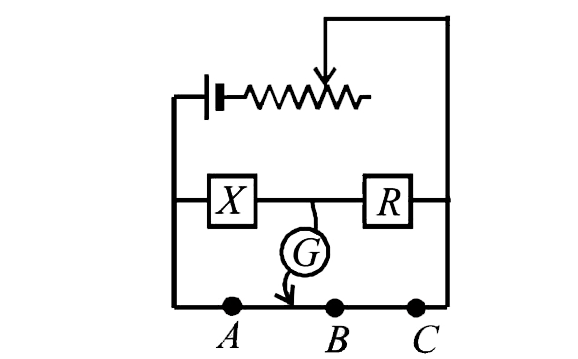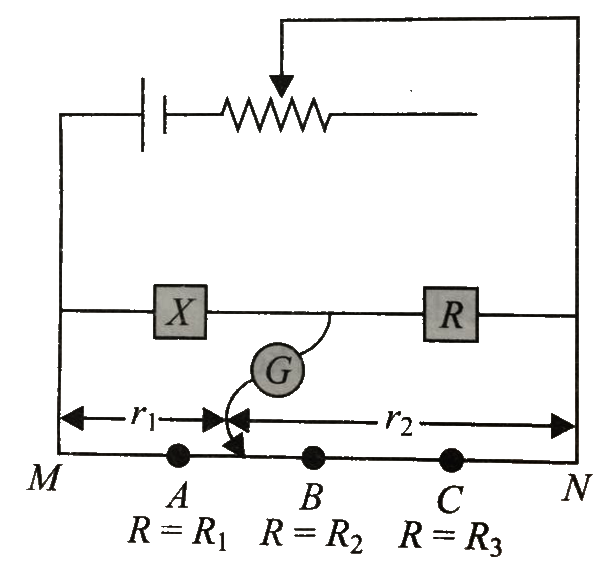Doubtnut App - Get 50 Lakh+ video solutions better than GoogleInstall Now

# An unknown resistance X is to be determined using resistances R1,R2orR3. Their corresponding null points are A, B and C. Find which of the above will give the most accurate reading and why?. R=R1orR2orR3.

Text Solution
Verified by Experts

## At all null points, the wheatstone bridge will be balanced. Therefore, Xr1=Rr2 or X=Rr1r2 where R is aconstant and R1 and r2 are varibles. The maximum faction error is ΔXX=Δr1r1+(Δr2)(r2) Here Δr1=Δr2=y (say), then ΔXX=y[r2+r1r1r2] For ΔX/X to be minimum, r1×r2 should be maximum [sincer1+r2=c(constant)]. Let E=r1×r2=r1×(c−r1) =r1c−r21 ∴dEdr1=c−2r1=0 or r1=c2 or r2=c2 or r1=r2 Thus, R2 gives the most accurate value.